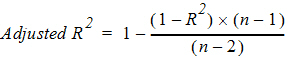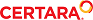﻿ Lambda Z or Slope Estimation settings

Lambda Z or Slope Estimation settings

This section pertains to NCA and IVIVC objects.

Phoenix will attempt to estimate the rate constant, Lambda Z, associated with the terminal elimination phase for concentration data. If Lambda Z is estimable, parameters for concentration data will be extrapolated to infinity. For NCA drug effect models, Phoenix estimates the two slopes at the begin­ning and end of the data. NCA does not extrapolate beyond the observed data for drug effect models.

Lambda Z or slope range selection

Phoenix will automatically determine the data points to include in Lambda Z or slope calculations as follows. (The exception to this is if the time range to use in the calculation of Lambda Z or slopes is not specified in an NCA object and curve stripping is not disabled, as described under “Options tab”.)

For concentration data: To estimate the best fit for Lambda Z, Phoenix repeats regressions of the natural logarithm of the concentration values using the last three points with non-zero concen­trations, then the last four points, last five points, etc. Points with a concentration value of zero are not included since the logarithm cannot be taken. Points prior to Cmax, points prior to the end of infusion, and the point at Cmax for non-bolus models, are not used in the Best Fit method (they can only be used if the user specifically requests a time range that includes them). For each regression, an adjusted R2 is computed:where n is the number of data points in the regression and R2 is the square of the correlation coefficient.

Lambda Z is estimated using the regression with the largest adjusted R2 and:
– If the adjusted R2 does not improve, but is within 0.0001 of the largest adjusted R2 value, the regression with the larger number of points is used.
– Lambda Z must be calculated from at least three data points.
– The estimated slope must be negative, so that its negative Lambda Z is positive.

For sparse sampling data: For sparse data, the mean concentration at each time (plasma or serum data) or mean rate for each interval (urine data) is used when estimating Lambda Z. Other­wise, the method is the same as for concentration data.

For drug effect data: Phoenix will compute the best-fitting slopes at the beginning of the data and at the end of the data using the same rules that are used for Lambda Z (best adjusted R-square with at least three points), with the exception that linear or log regression can be used according to the user's choice and the estimated slope can be positive or negative. If the user specifies the range only for Slope1, then in addition to computing Slope1, the best-fitting slope for Slope 2 will be computed at the end of the data. If the user specifies the range only for Slope2, then the best-fitting slope for Slope 1 will be computed at the beginning of the data.

The data points included in each slope are indicated on the Summary table of the output work­book and text for model 220. Data points for Slope1 are marked with “1” in workbook output and footnoted using “#” in text output; data points for Slope2 are labeled “2” and footnoted using an asterisk, “*”.

Calculation of Lambda Z

Once the time points being used in the regression have been determined either from the Best Fit method or from the user’s specified time range, Phoenix can estimate Lambda Z by performing a regression of the natural logarithm of the concentration values in this range of sampling times. The estimate slope must be negative, Lambda Z is defined as the negative of the estimated slope.

Note:Using this methodology, Phoenix will almost always compute an estimate for Lambda Z. It is the user’s responsibility to evaluate the appropriateness of the estimated value.

Calculation of slopes for effect data

Phoenix estimates slopes by performing a regression of the response values or their natural logarithm depending on the user’s selection. The actual slopes are reported; they are not negated as for Lambda Z.

Limitations of Lambda Z and slope estimation

It is not possible for Phoenix to estimate Lambda Z or slope in the following cases.

There are only two non-missing observations and the user requested automatic range selec­tion (Best Fit).

The user-specified range contains fewer than two non-missing observations.

Automatic range selection is chosen, but there are fewer than three positive concentration values after the Cmax of the profile for non-bolus models and fewer than two for bolus models or the slope is not negative.

For infusion data, automatic range selection is chosen, and there are fewer than three points at or after the infusion stop time.

The time difference between the first and last data points in the estimation range used is approximately < 1e –10.

In these instances, the curve fit for that subject will be omitted. The parameters that do not depend on Lambda Z (i.e., Cmax, Tmax, AUClast, etc.) will still be reported, but the software will issue a warning in the text output, indicating that Lambda Z was not estimable.